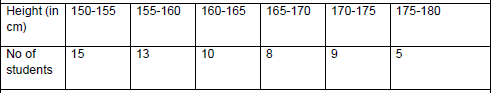# CBSE Class 10 Mathematics Standard Boards 2020 Sample Paper Solved

Read and download PDF of CBSE Class 10 Mathematics Standard Boards 2020 Sample Paper Solved designed as per the latest curriculum and examination pattern for Class 10 issued by CBSE, NCERT and KVS. The latest Class 10 Mathematics Sample Papers have been provided with solutions so that the students can solve these practice papers and then compare their answers. This will help them to identify mistakes and improvement areas in Mathematics Standard 10 which they need to study more to get better marks in Grade 10 exams. After solving these guess papers also refer to solved Class 10 Mathematics Question Papers available on our website to build strong understanding of the subject

## Mathematics Sample Paper Class 10 2020

Students can refer to the below Class 10 Mathematics Sample Paper designed to help students understand the pattern of questions that will be asked in Grade 10 exams. Please download CBSE Class 10 Mathematics Standard Boards 2020 Sample Paper Solved

### Mathematics Class 10 Sample Paper 2020

CBSE Class 10 Mathematics Standard Boards 2020 Sample Paper Solved. CBSE has recently released the sample papers for class 10 for subject Maths These papers are released every year by CBSE so that the students can understand the pattern of question paper which is expected to come in the Class 10 board exams. Students can refer to the attached question paper along with marking scheme to understand what they can expect in the board exams in 2020. Its always better to solve the question paper first and then refer to provided answers.

Q 1- Q 10 are multiple choice questions. Select the most appropriate answer from the given options

1. Consider the following frequency distribution of the heights of 60 students of a classThe upper limit of the median class in the given data is
a) 165
b) 155
c) 160
d) 170

2. The LCM of smallest two digit composite number and smallest composite number is
a) 12
b) 4
c) 20
d) 44

3. For which value(s) of p, will the lines represented by the following pair of linear equations be parallel
3x − y − 5 = 0
6x − 2y − p = 0
a) all real values except 10
b) 10
c) 5/2
d) 1/2

4. The point which divides the line segment joining the points (8, – 9) and (2, 3) in ratio 1 : 2 internally lies in the

5.The distance of the point P (−3, −4) from the x-axis (in units) is
a) 3
b) −3
c) 4
d) 5

6 The perimeters of two similar triangles ΔABC and ΔPQR are 35cm and 45cm respectively, then the ratio of the areas of the two triangles is______________

7 Fill the two blanks in the sequence 2, ____ , 26, ____ so that the sequence forms an A.P

8 A number is chosen at random from the numbers -5, -4, -3, -2, -1, 0, 1, 2, 3, 4, 5. Then the probability that square of this number is less than or equal to 1 is _____________

9 Write one rational and one irrational number lying between 0.25 and 0.32

10 If the angle between two tangents drawn from an external point ‘P’ to a circle of radius ‘r’ and centre O is 600, then find the length of OP.
OR
If the radii of two concentric circles are 4 cm and 5 cm, then find the length of each chord of one circle which is tangent to the other circle.

11 If the first three terms of an A.P are b, c and 2b, then find the ratio of b and c

12 Find the number of natural numbers between 102 and 998 which are divisible by 2 and 5 both.

13 Prove that the rectangle circumscribing a circle is a square.

14 Jayanti throws a pair of dice and records the product of the numbers appearing on the dice. Pihu throws 1 dice and records the squares the number that appears on it. Who has the better chance of getting the number 36? Justify?
OR
An integer is chosen between 70 and 100, Find the probability that it is
(a) a prime number
(b) divisible by 7

28 If the sum of first m terms of an AP is the same as the sum of its first n terms, show that the sum of its first (m+n) terms is zero.

## Tags:

Click for more Mathematics Study Material

## Latest NCERT & CBSE News

Read the latest news and announcements from NCERT and CBSE below. Important updates relating to your studies which will help you to keep yourself updated with latest happenings in school level education. Keep yourself updated with all latest news and also read articles from teachers which will help you to improve your studies, increase motivation level and promote faster learning

### CBSE Term 2 Board Examinations

CBSE vide Circular No.Acad-51/2021 dated 5th July, 2021, notified that in the session 2021-2022, Board Examinations would be conducted in two terms, i.e.. Term I and Term II. This decision was taken due to the uncertainty arising out of COVID 19 Pandemic. Term I...

### Board Exams Date Sheet Class 10 and Class 12

Datesheet for CBSE Board Exams Class 10  (Scroll down for Class 12 Datesheet) Datesheet for CBSE Board Exams Class 12

### Celebration of Matribhasha Diwas Mother Language day

UNESCO has declared 21st February of every year to be celebrated as International Mother Language day to promote dissemination of Mother Language of all, create awareness of linguistic and cultural traditions and diversity across the world and to inspire solidarity...

### Class 10th and 12th Term 2 Revaluation Process 2022

Evaluation of the Answer Books is done under a well-settled Policy. To ensure that the evaluation is error free, CBSE is taking several steps. After strictly following these steps, the result is prepared. Though, CBSE is having a well-settled system of assessment,...